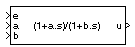Implement first-order lead-lag with gain-scheduled coefficients

• Library:
• Aerospace Blockset / GNC / Control

•## Description

The Gain Scheduled Lead-Lag block implements a first-order lag of the form

`$u=\frac{1+as}{1+bs}e$`

where e is the filter input, and u is the filter output.

The coefficients a and b are inputs to the block. These values can depend on the flight condition or operating point. For example, you can produce them from the Lookup Table (n-D) Simulink® block.

## Ports

### Input

expand all

Filter input, specified as a scalar.

Data Types: `single` | `double` | `int8` | `int16` | `int32` | `uint8` | `uint16` | `uint32` | `Boolean` | `fixed point` | `enumerated` | `bus`

Numerator coefficient, specified as a scalar.

Data Types: `single` | `double` | `int8` | `int16` | `int32` | `uint8` | `uint16` | `uint32` | `Boolean` | `fixed point` | `enumerated` | `bus`

Denominator coefficient, specified as a positive scalar.

Data Types: `single` | `double` | `int8` | `int16` | `int32` | `uint8` | `uint16` | `uint32` | `Boolean` | `fixed point` | `enumerated` | `bus`

### Output

expand all

Filter output, specified as a scalar.

Data Types: `single` | `double` | `int8` | `int16` | `int32` | `uint8` | `uint16` | `uint32` | `Boolean` | `fixed point` | `enumerated` | `bus`

## Parameters

expand all

Initial internal state, specified as a vector, for the filter `x_initial`. Given this initial state, the initial output is given by

`${u|}_{t=0}=\frac{x_initial+ae}{b}$`

#### Programmatic Use

 Block Parameter: `initial state, x_initial` Type: character vector Values: vector Default: `'0'`

## Extended Capabilities

### C/C++ Code GenerationGenerate C and C++ code using Simulink® Coder™.